0%

《Input Convex Neural Networks》

《Optimal Control Via Neural Networks: A Convex Approach》

1. 复现ICNN，原版的ICNN是用tensorflow写的，代码不全，且使用了较老的库版本，运行不了，在此采用pytorch进行复现出一个通用程序。
2. 对于一个给定的函数：$$y=3x_1^2+4x_2^2$$，利用普通的DNN，和ICNN进行对比，验证ICNN的拟合能力（验证的过程使用相同的网络层数，每层的节点数，学习率等参数）。
3. 对于《Input Convex Neural Networks》中的simulation中的synthetic 2D example和《Optimal Control Via Neural Networks: A Convex Approach》中的APPENDIX中的Toy Example分别进行复现及仿真验证。

## ICNN原理及证明

### ICNN网络结构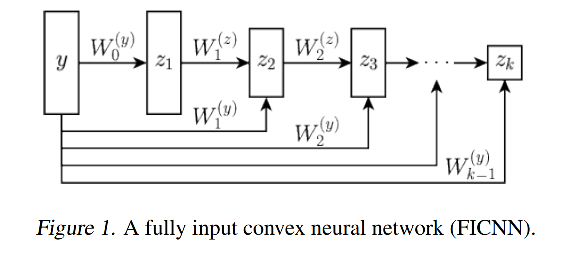$z_{i+1}=g_{i}\left(W_{i}^{(z)} z_{i}+W_{i}^{(y)} y+b_{i}\right), \quad f(y ; \theta)=z_{k}$

Figure1是全连接的凸的，k层ICNN，记为：FICNN，令输入记为：$$y$$$$z_i$$记为每一个激活函数的输出，$$\theta$$记为所有的参数：$$\theta=\left\{W_{0: k-1}^{(y)}, W_{1: k-1}^{(z)}, b_{0: k-1}\right\}$$$$g_i$$是非线性激活函数，网络凸的性质的主要结果如下：

1. ICNN网络结构中，从输入直接传递到隐含层的叫"passthrough"，这样的连接在传统的前馈神经网络中是没有必要的，因为前面的神经网络的层的信息总是会被映射到下一个神经网络层中，但是在ICNN中，由于$$W^{(z)}$$被限制为非负，所以导致拟合能力下降，且前面网络的信息无法被传递到下一个层中，因此，需要使用额外的passthrough。

2. 对于$$b_i$$来说，放到前向传播中，或者passthrough中，都是等价的。

3. passthrough中的weight和bias都是可以为负的，没有限制。

### ICNN输入凸的证明

1. 凸函数的非负先行组合还是凸函数
2. 凸函数和凸的非减的函数还是凸函数

$f_{C P L}(\mathbf{x})=\max \left\{\mathbf{a}_{1}^{T} \mathbf{x}+b_{1}, \mathbf{a}_{2}^{T} \mathbf{x}+b_{2}\right\}$

$f_{C P L}(x)=\left(\mathbf{a}_{2}^{T} \mathbf{x}+b_{2}\right)+\max \left(\left(\mathbf{a}_{1}-\mathbf{a}_{2}\right)^{T} \mathbf{x}+\left(b_{1}-b_{2}\right), 0\right)$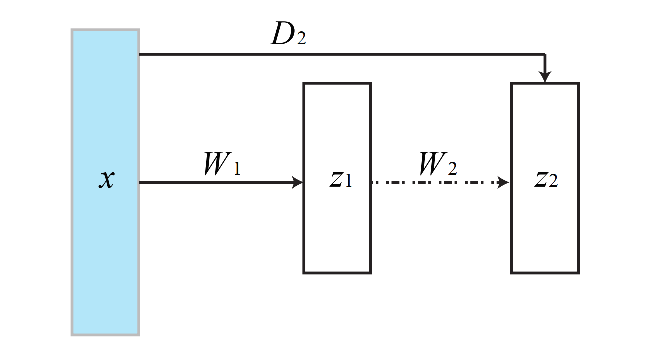$f_{C P L}(\mathbf{x})=\max \left\{\mathbf{a}_{1}^{T} \mathbf{x}+b_{1}, \ldots, \mathbf{a}_{K}^{T} \mathbf{x}+b_{K}\right\}$

$$f_{C P L}(\mathbf{x})$$写成一个嵌套的最大值的仿射函数的最大值，为了简化表示，将$$L_{i}=\mathbf{a}_{i}^{T} \mathbf{x}+b_{i}, L_{i}^{\prime}=L_{i}-L_{i+1}$$，然后： \begin{aligned} f_{C P L} &=\max \left\{L_{1}, L_{2}, \ldots, L_{K}\right\} \\ &=\max \left\{\max \left\{L_{1}, L_{2}, \ldots, L_{K-1}\right\}, L_{K}\right\} \\ &=L_{K}+\sigma\left(\max \left\{L_{1}, L_{2}, \ldots, L_{K-1}\right\}-L_{K}\right) \\ &=L_{K}+\sigma\left(\max \left\{\max \left\{L_{1}, L_{2}, \ldots, L_{K-2}\right\}, L_{K-1}\right\}-L_{K}, 0\right) \\ &=L_{K}+\sigma\left(L_{K-1}-L_{K}+\sigma\left(\max \left\{L_{1}, L_{2}, \ldots, L_{K-2}\right\}-L_{K-1}, 0\right), 0\right) \\ &=\ldots \\ &=L_{K}+\sigma\left(L_{K-1}^{\prime}+\sigma\left(L_{K-2}^{\prime}+\sigma\left(\ldots \sigma\left(L_{2}^{\prime}+\sigma\left(L_{1}-L_{2}, 0\right), 0\right), \ldots, 0\right), 0\right), 0\right) . \end{aligned} 最后一行表示的其实是一个$$K$$层的神经网络，其每一层分别是： \begin{aligned} &z_{1}=\sigma\left(L_{1}-L_{2}, 0\right)=\sigma\left(\left(\mathbf{a}_{1}-\mathbf{a}_{2}\right)^{T} \mathbf{x}+\left(b_{1}-b_{2}\right)\right)\\ &z_{2}=\sigma\left(L_{2}^{\prime}+z_{1}, 0\right)=\sigma\left(z_{1}+\left(\mathbf{a}_{2}-\mathbf{a}_{3}\right)^{T} \mathbf{x}+\left(b_{2}-b_{3}\right)\right)\\ &\ldots \ldots\\ &z_{i}=\sigma\left(L_{i}^{\prime}+z_{i-1}, 0\right)=\sigma\left(z_{i-1}+\left(\mathbf{a}_{i}-\mathbf{a}_{i+1}\right)^{T} \mathbf{x}+\left(b_{i}-b_{i+1}\right)\right)\\ &\ldots \ldots\\ &z_{K}=z_{K-1}+L_{K}=h_{K}\left(z_{K-1}+L_{K}\right)=\left(z_{K-1}+\mathbf{a}_{K}^{T} \mathbf{x}+b_{K}\right) \end{aligned} 尽管该神经网络可以用来表示仿射函数的最大值，但是它不是凸的，因为其系数可正可负，而且，每一层都包含了一个权重和输入的内积 $$\left(\mathbf{a}_{i}-\mathbf{a}_{i+1}\right)^{T} \mathbf{x}$$，系数未必是非负的，为了解决这个问题，将输入$$x$$扩展为$$[x, -x]$$，即： $\hat{\mathbf{x}}=\left[\begin{array}{c} \mathbf{x} \\ -\mathbf{x} \end{array}\right]$ $$\mathbf{h}^{T} \mathbf{x}$$的内积可以表示为： \begin{aligned} \mathbf{h}^{T} \mathbf{x} &=\sum_{j=1}^{d} h_{i} x_{i} \\ &=\sum_{i: h_{i} \geq 0} h_{i} x_{i}+\sum_{i: h_{i}<0} h_{i} x_{i} \\ &=\sum_{i: h_{i} \geq 0} h_{i} x_{i}+\sum_{i: h_{i}<0}\left(-h_{i}\right)\left(-x_{i}\right) \\ &=\sum_{i: h_{i} \geq 0} h_{i} \hat{x}_{i}+\sum_{i: h_{i}<0}\left(-h_{i}\right)\left(\hat{x}_{i+d}\right) \end{aligned} 其所有的系数都是非负的。

## $$y=3x_1^2+4x_2^2$$仿真算例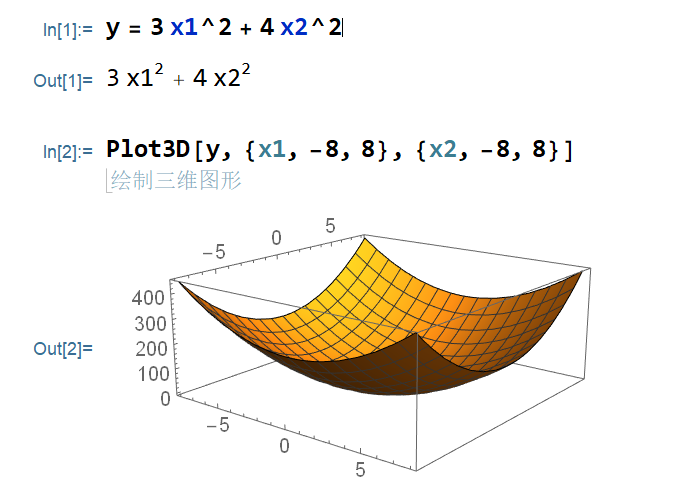### 对比其拟合能力：

#### 普通神经网络的拟合能力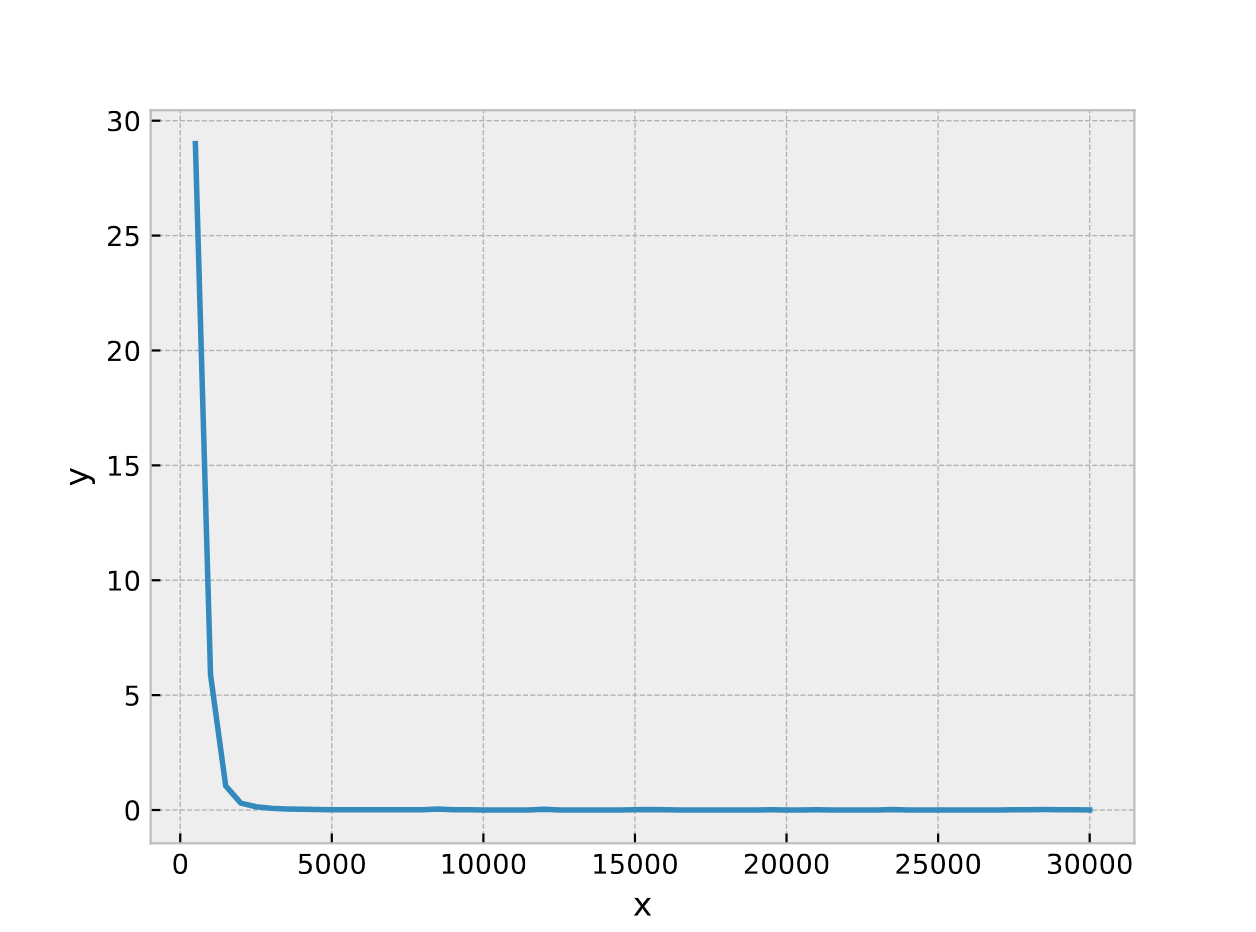#### ICNN的拟合能力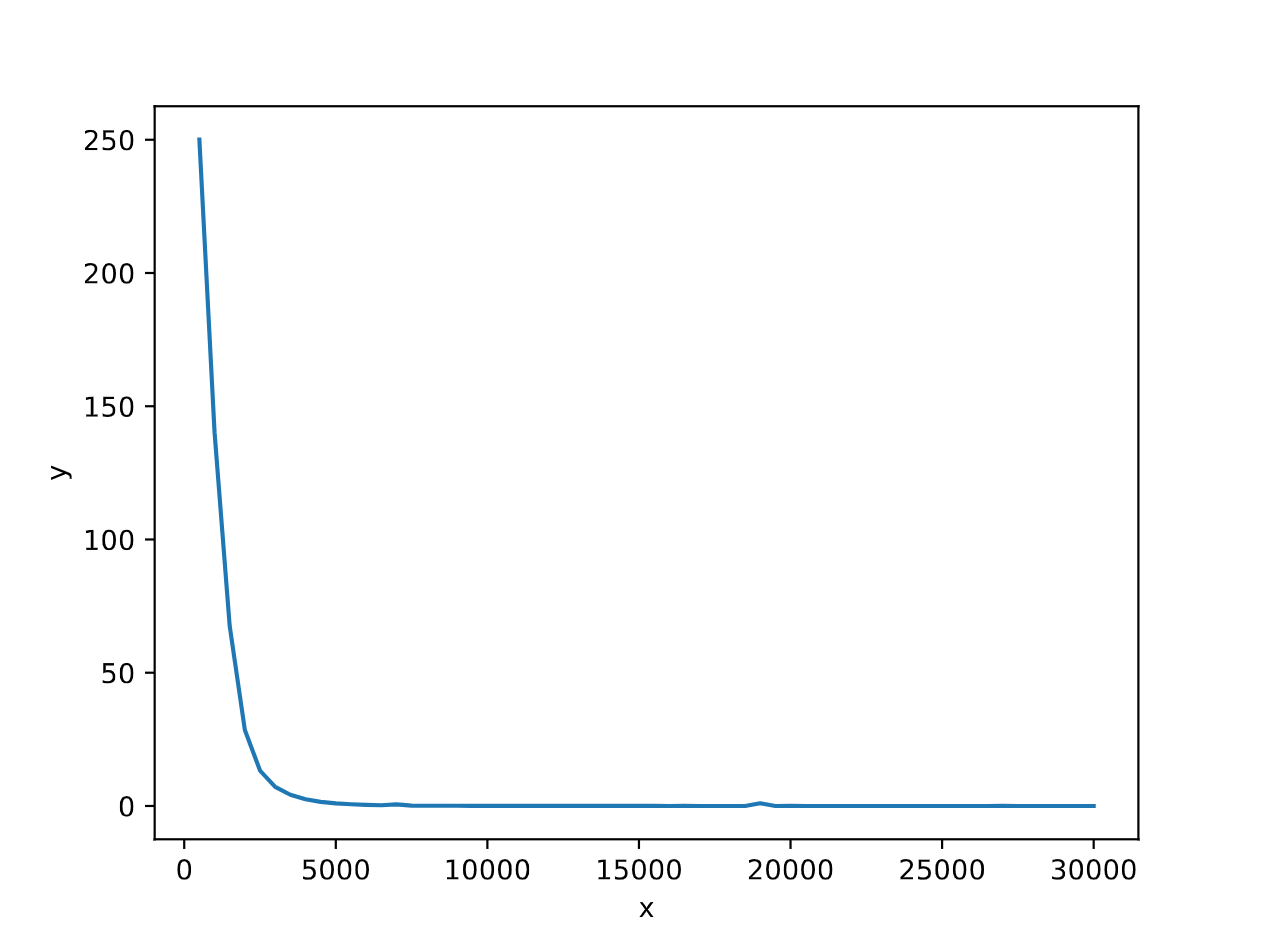## 原始论文中的仿真验证的复现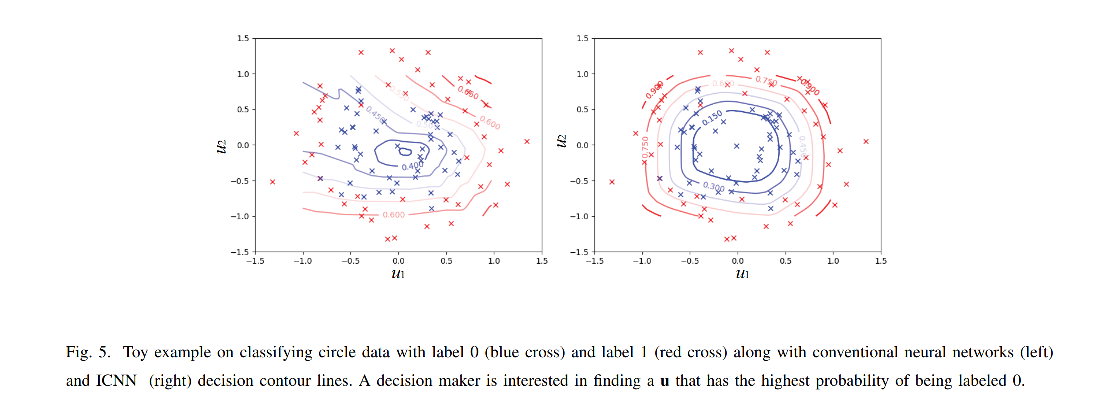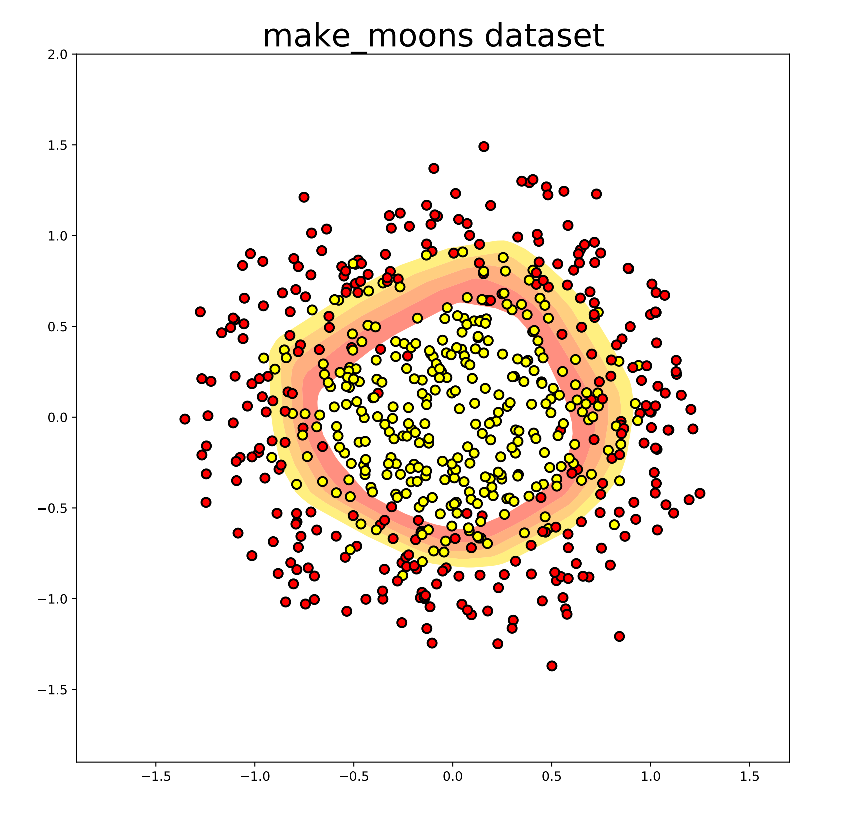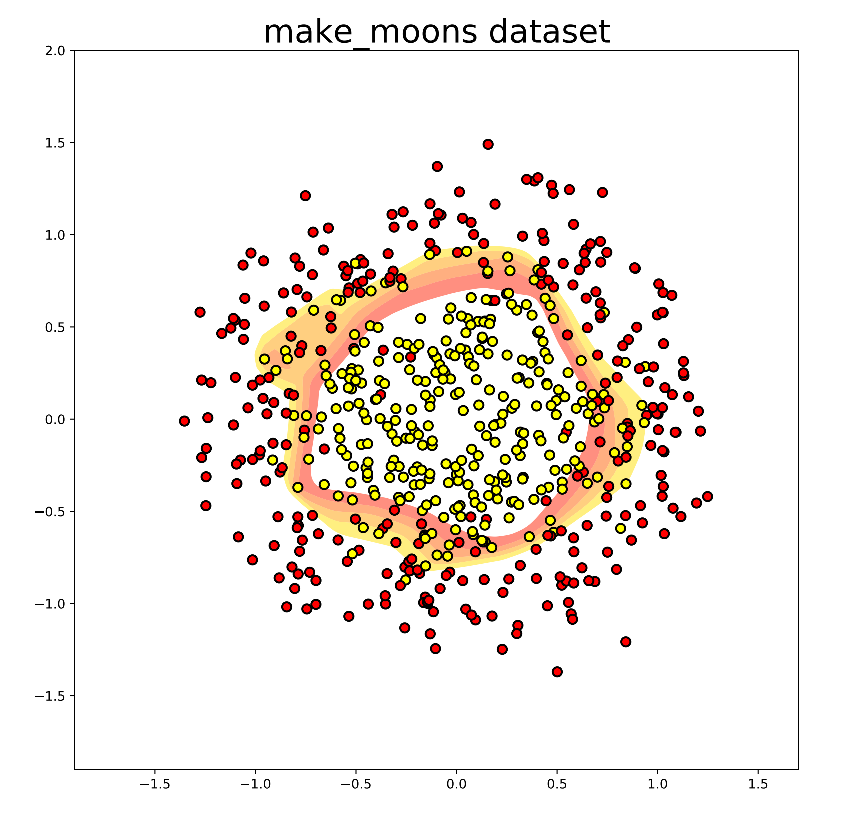## 未来展望

ICNN是一种2016年提出来的新型的神经网络结构，用于解决传统的神经网络难以inference的问题，同时，经过仿真验证，ICNN的拟合能力损失不大。不过经过检索，使用ICNN结构的发表在期刊上只有几篇文章，有很多篇都是发表在了会议上(ICML)，主要都是将ICML结合到特定的应用场景中。

《Data-Driven Solution for Optimal Pumping Units Scheduling of Smart Water Conservancy， 2020》这篇文章中作者在最后的展望中提到了”Additional effort is also needed to develop data-driven or learning-based models with convex characteristics of input–output dynamic relation, e.g., the input convex neural network that is able to model the convex optimization problem and obtain the optimal control solutions.“

If you like my blog, please donate for me.